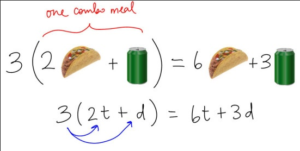## Simplifying Compound Expressions With Real Numbers

### Learning Outcomes

• Simplify expressions with fraction bars, brackets, and parentheses
• Simplify expressions using the distributive property
• Use the distributive property to simplify expressions with multiple grouping symbols

In this section, we will use the skills from the last section to simplify mathematical expressions that contain many grouping symbols and many operations. We are using the term compound to describe expressions that have many operations and many grouping symbols. More care is needed with these expressions when you apply the order of operations. Additionally, you will see how to handle absolute value terms when you simplify expressions.

### Example

Simplify $\frac{5-[3+(2\cdot (-6))]}{{{3}^{2}}+2}$

The video that follows contains an example similar to the written one above. Note how the numerator and denominator of the fraction are simplified separately.

## Absolute Value

Absolute value expressions are one final method of grouping that you may see. Recall that the absolute value of a quantity is always positive or $0$.

### Try It

When you see an absolute value expression included within a larger expression, treat the absolute value like a grouping symbol and evaluate the expression within the absolute value sign first. Then take the absolute value of that expression. The example below shows how this is done.

### Example

Simplify $\frac{3+\left|2-6\right|}{2\left|3\cdot1.5\right|-\left(-3\right)}$.

The following video uses the order of operations to simplify an expression in fraction form that contains absolute value terms. Note how the absolute values are treated like parentheses and brackets when using the order of operations.

## The Distributive Property

Parentheses are used to group or combine expressions and terms in mathematics.  You may see them used when you are working with formulas, and when you are translating a real situation into a mathematical problem so you can find a quantitative solution.Combo Meal Distributive Property

For example, you are on your way to hang out with your friends, and call them to ask if they want something from your favorite drive-through.  Three people want the same combo meal of $2$ tacos and one drink. You can use the distributive property to find out how many total tacos and how many total drinks you should take to them.

$\begin{array}{c}\,\,\,3\left(2\text{ tacos }+ 1 \text{ drink}\right)\\=3\cdot{2}\text{ tacos }+3\text{ drinks }\\\,\,=6\text{ tacos }+3\text{ drinks }\end{array}$

The distributive property allows us to explicitly describe a total that is a result of a group of groups. In the case of the combo meals, we have three groups of ( two tacos plus one drink). The following definition describes how to use the distributive property in general terms.

### The Distributive Property of Multiplication

For all real numbers a, b, and c, $a(b+c)=ab+ac$.
What this means is that when a number multiplies an expression inside parentheses, you can distribute the multiplication to each term of the expression individually.

To simplify  $3\left(3+y\right)-y+9$, it may help to see the expression translated into words:

multiply $3$ by (the sum of three and $y$), then subtract $y$, then add $9$

To multiply three by the sum of three and $y$, you use the distributive property –

$\begin{array}{c}\,\,\,\,\,\,\,\,\,3\left(3+y\right)-y+9\\\,\,\,\,\,\,\,\,\,=\underbrace{3\cdot{3}}+\underbrace{3\cdot{y}}-y+9\\=9+3y-y+9\end{array}$

Now you can subtract $y$ from $3y$ and add $9$ to $9$.

$\begin{array}{c}9+3y-y+9\\=18+2y\end{array}$

The next example shows how to use the distributive property when one of the terms involved is negative.

### Example

Simplify $a+2\left(5-a\right)+3\left(a+4\right)$

## Contribute!

Did you have an idea for improving this content? We’d love your input.